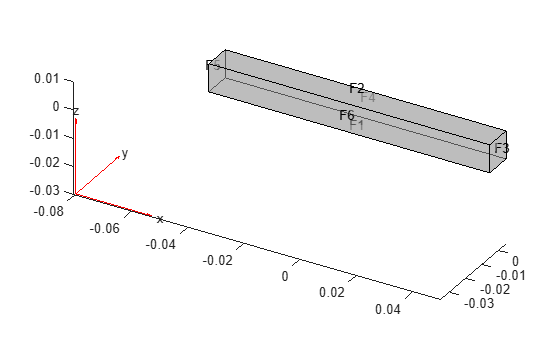Main Content

# reduce

Reduce structural model

## Syntax

``Rcb = reduce(structuralmodel,'FrequencyRange',[omega1,omega2])``

## Description

example

````Rcb = reduce(structuralmodel,'FrequencyRange',[omega1,omega2])` reduces a structural analysis model to the fixed interface modes in the frequency range `[omega1,omega2]` and the boundary interface degrees of freedom.```

## Examples

collapse all

Reduce the model to the fixed interface modes in the specified frequency range and the boundary interface degrees of freedom.

Create a transient structural model for a 3-D problem.

`structuralmodel = createpde('structural','transient-solid');`

Create a geometry and include it in the model. Plot the geometry.

```gm = multicuboid(0.1,0.01,0.01); structuralmodel.Geometry = gm; pdegplot(structuralmodel,'FaceLabels','on','FaceAlpha',0.5)```Specify the Young's modulus, Poisson's ratio, and mass density of the material.

```structuralProperties(structuralmodel,'YoungsModulus',70E9, ... 'PoissonsRatio',0.3, ... 'MassDensity',2700);```

Generate a mesh.

`generateMesh(structuralmodel);`

Specify the ends of the beam as structural superelement interfaces. The reduced-order model technique retains the degrees of freedom on the superelement interfaces while condensing the degrees of freedom on all other boundaries. For better performance, use the set of edges that bound each side of the beam instead of using the entire face.

```structuralSEInterface(structuralmodel,'Edge',[4,6,9,10]); structuralSEInterface(structuralmodel,'Edge',[2,8,11,12]);```

Reduce the model to the fixed interface modes in the frequency range `[-Inf,500000]` and the boundary interface degrees of freedom.

`R = reduce(structuralmodel,'FrequencyRange',[-Inf,500000])`
```R = ReducedStructuralModel with properties: K: [166x166 double] M: [166x166 double] NumModes: 22 RetainedDoF: [144x1 double] ReferenceLocations: [] Mesh: [1x1 FEMesh] ```

## Input Arguments

collapse all

Structural model, specified as a `StructuralModel` object. The model contains the geometry, mesh, structural properties of the material, body loads, boundary loads, and boundary conditions.

Example: ```structuralmodel = createpde('structural','transient-solid')```

Frequency range, specified as a vector of two elements. Define `omega1` as slightly smaller than the lowest mode's frequency and `omega2` as slightly larger than the highest mode's frequency. For example, if the lowest expected frequency is zero, then use a small negative value for `omega1`.

You can find natural frequencies and mode shapes for the specified frequency range by solving a modal analysis problem first. Then you can use a more precise frequency range to reduce the model. Note that a modal analysis problem still requires you to specify a frequency range. For example, see Modal Superposition Method for Structural Dynamics Problem.

Example: `[-0.1,1000]`

Data Types: `double`

## Output Arguments

collapse all

Structural results obtained using the Craig-Bampton order reduction method, returned as a `ReducedStructuralModel` object.

## See Also

Introduced in R2019b

## SupportGet trial now